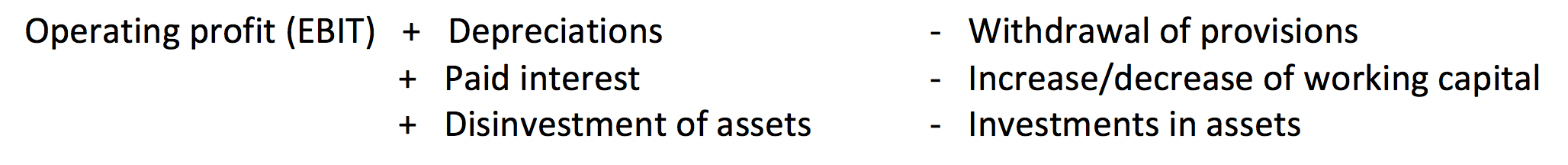Self Help Documentation

# Discounted Cash Flow

Whether you want to sell your online business, to acquire another business or just want to investigate how you stand: knowing your online business value is interesting at any time. The discounted cash flow method (DCF) is one of the most widely used methods to determine business value.

The DCF is praised for not only looking at the historical value of a company, but also for future free cash flows. This also makes the future value insightful. And the future of a business is exactly what it’s all about for a buyer in an acquisition.

### Future cash flow determines the market value of your online business

An easy way to determine the value of an online business is based on the so-called intrinsic value. This is the total of all the assets of the company, less its debt. However, it is more interesting to look at the future, rather than the current value. What earnings can the web store generate in the future? That market value is calculated by using the DCF method. Hereby, you appreciate the future cash flows and make this cash (converted to the current value) by calculating what costs you must make to actually earn those cash flows.

The reasoning behind this is that ‘current money’ has more value than ‘future money’. Think of it like this: would you rather have 200 euros in your pocket right now or would you rather receive the 200 euros in one year, considering all risks involved that you’ll never see that money?  In other words, the future 200 euros has less value today than the 200 euros that’s currently in your pocket. How much less, that depends on several (partly assumed) risk factors that we will further explain on this page.

(The difference between the market value (calculated with the DFC) and the intrinsic value is also referred to as goodwill!)

### Determine online business value

To calculate the value of an online business using the Discounted Cash Flow method, you’ll need to consider 3 factors: Time, Money and Risk. These are reflected in two variables: The expected annual free cash flow & The cost of invested assets.

#### 1.The expected annual free cash flow

The free cash flow means all expected income and outflow of cash flow in a certain period. It’s the total that remains or that a web store has short, after all the necessary investments have been made to ensure continuity. In other words, the free cash flow represents the market value of a company.

There are three different types of cash flow:

– Operational cash flow
– Cash flow from investments
– Financing cash flow

The free cash flow is the cash flow that is attributable to the providers of equity and debt. Hence, this is the sum of operating cash flow and cash flow from investments.

In a formula, this can be displayed as follows:

####2.The cost of capital invested:

In order to understand the company value, future cash flows must be related to the cost to make it ‘cash’. This is done by calculating the average cost of the invested capital. This invested capital consists of the sum of equity and debt.

The cost of the debt is fairly easy to calculate: it’s the average interest rate that is paid on short-term and long-term debt.

The cost of equity is calculated using the risk-free interest rate (“Rf”), plus an investment risk (Rm) and a premium for investing in a small company (‘Rs’), the so-called ‘small firm premium’ (used as a ‘online business premium’ in our case) and a possible company-specific risk factor (‘Ru’).

For example, the required yield might look like this: 2% (risk-free interest rate) + 5.5% (historical return on shares) + 12.5% ​​(online business premium) + 11% (specific risk factor for the web store in question) = 31% .

Cost of total assets

When the costs of equity and debt are known, these should be converted to the cost of total assets. To this end, you calculate the ratio between equity and debt. For example, suppose a web store has been funded with 15% of loaned capital, then the average cost of capital can be calculated as following (based on the above-mentioned required yield of 31% and an average cost of external capital of 6%).

(31% *0.85) + (6% * 0.15)/100 = 27.25%